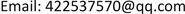1国网辽宁省电力有限公司，辽宁 沈阳

2国网辽宁省电力有限公司沈阳供电公司，辽宁 沈阳1. 引言

2. 辽宁省电力景气先行指数2.1. 辽宁省电力景气指标的筛选

2.2. 辽宁省电力景气先行指数的编制

Preliminary indicator factor composition tabl

L = 0.784 x 1 − 0.803 x 2 + 0.727 x 3 (4-19)

Liaoning power boom first synthetic index tabl

2013(1)100.0002015(3)100.014
2013(2)101.4412015(4)99.901
2013(3)100.1842016(1)97.899
2013(4)100.0502016(2)101.729
2014(1)98.2642016(3)100.312
2014(2)101.6132016(4)98.679
2014(3)99.9992017(1)99.143
2014(4)99.8792017(2)101.042
2015(1)97.8592017(3)100.970
2015(2)101.6152017(4)100.468

3. 辽宁省电力景气先行指数波动分析

2013年第一季度至2017年第四季度期间，辽宁电力景气先行指数在每年的第一季度均达到该年景气指数最低值，即波谷；均在第二季度达到景气指数的最大值，即波峰；每年第三季度、第四季度的电力景气指数较为接近，除2016年第四季度先行指数较同年第三季度指数有较大下滑之外，其余年份第四季度较第三季度的指数均呈小幅下降趋势。虽然2017年景气先行指数波动状态出现偏差，即同比往年波峰2017年波峰指数降幅较大，但考虑到2017年其它季度的景气先行指数同比往年有较大幅度的升高，并且由于2013年至2016年期间，每年先行指数波峰均略高于前一年波峰，2013年至2017年期间，指数波谷于2015年达到最低值后开始呈现上升趋势，因此辽宁电力景气先行合成指数总体仍呈现出一种缓慢稳步攀升的态势。

4. 电力景气先行指数与用电需求相关性分析

4.1. 向量自回归(VAR)模型的建立

y t = A 1 y t − 1 + A 2 y t − 2 + ⋯ + A p y t − p + B x t + μ t ，或者是 A ( L ) y t = μ t ， t = 1 , 2 , 3 , ⋯ , T (5-5)

y t = C ( L ) μ t (5-6)

Σ = 1 T ∑ ​   μ ^ t μ ^ ′ t (5-7)

A I C = − 2 l T + 2 n / T , S C = − 2 l T + n ln T / T (5-8)

4.2. 单位根检验

Δ y t = γ y t − 1 + ∑ i = 1 p β i Δ y t − 1 + μ i , (5-8)

Δ y t = γ y t − 1 + α + ∑ i = 1 p β i Δ y t − 1 + μ i , t = 1 , 2 , ⋯ , T (5-9)

Δ y t = γ y t − 1 + α + α ˜ + ∑ i = 1 p β i Δ y t − 1 + μ i , t = 1 , 2 , ⋯ , T (5-10)

{ H 0 : γ = 0 H 1 : γ < 0 (5-11)

4.3. 格兰杰因果检验

H0：；H1：至少存在一个q使得 A 12 ( q ) ≠ 0 。

VAR回归模型存在着一个渐近等价检验，可由下式给出：

S 2 = T ( R S S O − R S S 1 ) R S S 1 ~ X 2 ( p ) (5-14)

S 2 服从自由度为p的 X 2 分布。如果 S 2 大于 X 2 的临界值，则拒绝原假设；否则接受原假设。

4.4. 脉冲响应函数分析

Y t + s = U t + s + A 1 U t + s − 1 + A 1 2 U t + s − 2 + ⋯ + A 1 s U t + ⋯ (5-15)

Y t + s = U t + s + Ψ 1 U t + s − 1 + Ψ 2 U t + s − 2 + ⋯ + Ψ s U t + ⋯ (5-16)

Ψ s = ∂ Y t + s ∂ U t (5-17)

4.5. 方差分解分析

y = ∑ j = 1 p ( Ψ 0 i j μ j t + Ψ 1 i j μ j t − 1 + Ψ 2 i j μ j t − 2 + Ψ 3 i j μ j t − 3 + ⋯ ) (5-18)

E [ ( Ψ 0 i j μ j t + Ψ 1 i j μ j t − 1 + Ψ 2 i j μ j t − 2 + Ψ 3 i j μ j t − 3 + ⋯ ) 2 ] = ∑ q = 0 ∞ ( Ψ q i j ) 2 σ i j 2 (5-19)

R V C j → i ( ∞ ) = ∑ q = 0 ∞ ( Ψ q i j ) 2 σ i j 2 ∑ j = 1 k { ∑ q = 0 ∞ ( Ψ q i j ) 2 σ i j 2 } (5-20)

5. 结论与建议